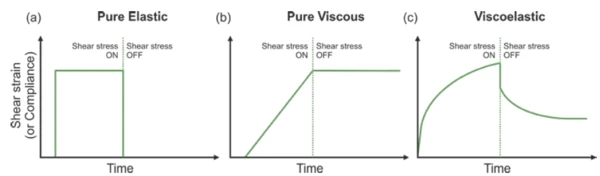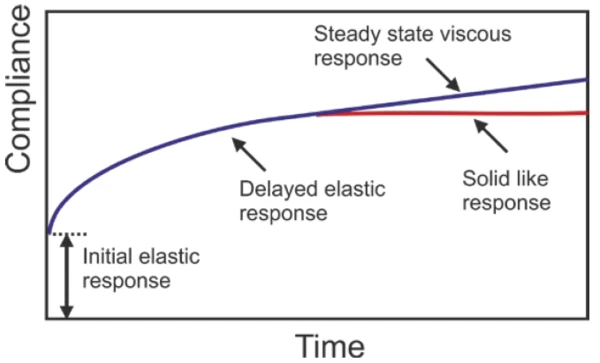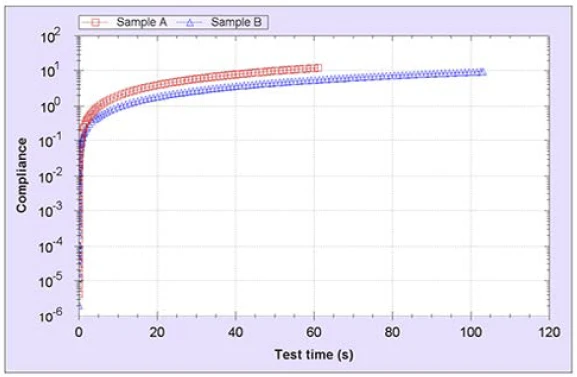# Assessing the Dispersion Stability Using Creep Testing on a Rotational Rheometer – Shower Gels

#### Introduction

Whether the long-term stability of an emulsion or suspension is governed by a zero shear viscosity or a Yield StressYield stress is defined as the stress below which no flow occurs; literally behaves like a weak solid at rest and a liquid when yielded.yield stress is dependent upon its microstructure. Furthermore, it is the state of this microstructure at long timescales that is important since this is ultimately what any dispersed phase will encounter over prolonged storage. One way to determine the existence, and if so magnitude of this low shear (zero shear) viscosity is from a creep test. This test involves applying a constant stress over a prolonged timescale and monitoring the resultant strain or compliance (strain/stress) as a function of time.

As shown in Figure 1, a purely viscous material will show a constant increase in compliance with time indicative of flow, i.e., steady state viscous response. In contrast, a solid will show a zero gradient indicating an elastic response.1) Principle of creep testing for an (a) elastic; (b) viscous; (c) viscoelastic materials

For viscoelastic materials, the response will be a combination of viscous and elastic effects as shown in Figure 2, with the response at long times indicating whether it has a Yield StressYield stress is defined as the stress below which no flow occurs; literally behaves like a weak solid at rest and a liquid when yielded.yield stress (elastic) or a steady state viscous response (viscous). If the material shows a steady-state viscous response, then the zero shear viscosity is simply given by the inverse gradient of the compliance versus time plot.2) Kriechen (Rheologie)Creep is one of the earliest “controlled stress” rheometer tests that quite literally “creeps” the material, i.e. we measure over a relatively prolonged period the small movement (the creep defined as creep compliance, J) of the sample by applying a small constant stress.Creep response for a viscoelastic liquid (blue) and a viscoelastic solid (red)

If the average particle size, density and volume fraction of the dispersed phase are known, and the zero shear viscosity of the continuous phase is determined, then the velocity (V) of the dispersed phase can be estimated using the following modification of Stokes equation:

a = the particle radius (sphere)
Δρ = the density difference between particle and liquid
η = the viscosity of the liquid (zero shear viscosity)
g = acceleration due to gravity
φ = the phase volume

The value of the exponent is generally 4.75 for a radius >1 μm and 5.25 for a radius <1 μm.

Potentially, the dispersion can then be re-formulated to obtain a low shear viscosity sufficient to suspend the dispersed phase for the required period, or alternatively to introduce a Yield StressYield stress is defined as the stress below which no flow occurs; literally behaves like a weak solid at rest and a liquid when yielded.yield stress which would give a solid-like response at long times.

It is important when running a creep test that sufficient time is allowed for steady state to be reached. If not, then too low a value of the zero shear viscosity may be reported or indeed an incorrect assumption is made that the material has a zero shear viscosity when it actually has a Yield StressYield stress is defined as the stress below which no flow occurs; literally behaves like a weak solid at rest and a liquid when yielded.yield stress. Since dispersions may sit on a shelf undisturbed for weeks or even months at a time, then this needs to be considered in any test protocol.

This application note shows methodology and data from creep testing for two commercial shower gel products.

#### Results and Discussion

Figure 3 shows the creep response for the two shower gel products on a logarithmic scale. Clearly the two products show a similar initial elastic response, however, there are differences in the delayed elastic response as indicated by the timescale required to achieve steady state. The steady state tolerance range employed in this test was within ± 1% for 60 seconds. Clearly sample A displays steady state behavior almost immediately, while Sample B has some residual elasticity.3) Plots of log compliance against test time for the two shower gel products

Figure 4 shows the same plot but using a linear scale. This makes it easier to distinguish the differences in the gradient of the compliance versus time plots. Since η0 is given by the inverse gradient of the steady state portion of the curve then it is clear that sample B will have a higher zero shear viscosity than sample A. These values were automatically calculated as part of the test and were found to be 6 Pas and 12 Pas for sample A and B, respectively. According to Equation 1, this means that sample B would reduce the rate of sedimentation by a factor of 2 for suspensions of equivalent particles and volume fractions. Whether these viscosity values are sufficient to give effective stability will depend on the specific size, density and volume of the dispersed phase, which need to be determined using independent techniques.